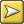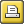﻿ p value
 p value(Default=0.000001)

The p value is that used in standard chi-square and other statistical tests. This value ranges from 0 to 1. A value of .01 suggests a 1% danger of being wrong in claiming a relationship, .05 would give a 5% danger of error. In the social sciences a 5% risk is usually considered acceptable.

In the case of key word analyses, where the notion of risk is less important than that of selectivity, you may often wish to set a comparatively low p value threshold such as 0.000001 (one in 1 million) (1E-6 in scientific notation) so as to obtain fewer key words. Or you can set a low "maximum wanted" number in the main Controller, under Adjust Settings | KeyWords.

If the chi-square procedure is used, the computed p value will only be shown if all appropriate statistical requirements are met (all expected values >= 5).